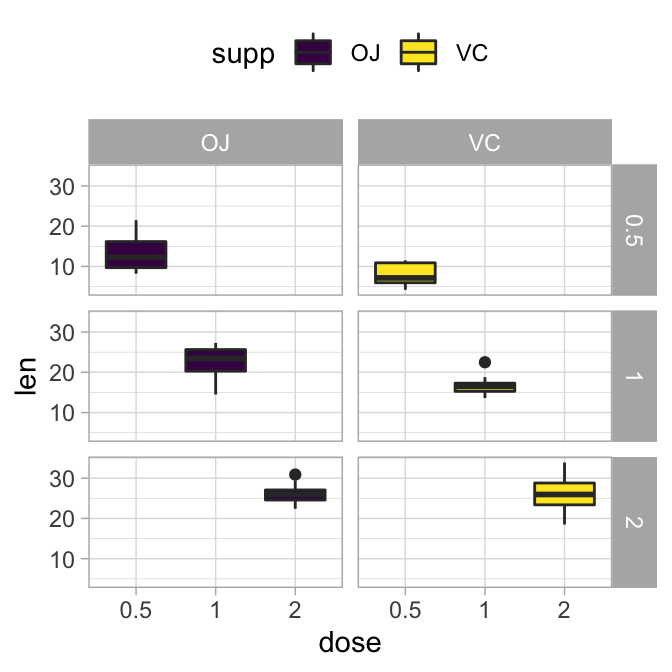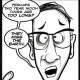# How to Change GGPlot Facet LabelsContents:

#### Related Book

GGPlot2 Essentials for Great Data Visualization in R

## Prerequisites

Load required packages and set the theme function theme_light() [ggplot2] as the default theme:

library(ggplot2)
theme_set(
theme_light() + theme(legend.position = "top")
)

## Basic ggplot with facet

Create a box plot filled by groups:

# Load data and convert dose to a factor variable
data("ToothGrowth")
ToothGrowth$dose <- as.factor(ToothGrowth$dose)

# Box plot, facet accordding to the variable dose and supp
p <- ggplot(ToothGrowth, aes(x = dose, y = len)) +
geom_boxplot(aes(fill = supp), position = position_dodge(0.9)) +
scale_fill_viridis_d()
p + facet_grid(dose ~ supp)## Change the text of facet labels

Facet labels can be modified using the option labeller, which should be a function.

• In the following R code, facets are labelled by combining the name of the grouping variable with group levels. The labeller function label_both is used.
p + facet_grid(dose ~ supp, labeller = label_both)• A simple way to modify facet label text, is to provide new labels as a named character vector:
# New facet label names for dose variable
dose.labs <- c("D0.5", "D1", "D2")
names(dose.labs) <- c("0.5", "1", "2")

# New facet label names for supp variable
supp.labs <- c("Orange Juice", "Vitamin C")
names(supp.labs) <- c("OJ", "VC")

# Create the plot
p + facet_grid(
dose ~ supp,
labeller = labeller(dose = dose.labs, supp = supp.labs)
)• An alternative solution to change the facet labels, is to modify the data:
df <- ToothGrowth
# Modify the data
df$dose <- factor(df$dose, levels = c("0.5", "1", "2"),
labels = c("D0.5", "D1", "D2"))
df$supp <- factor(df$supp, levels = c("OJ", "VC"),
labels = c("Orange Juice", "Vitamin C")
)
# Create the plot
ggplot(df, aes(x = dose, y = len)) +
geom_boxplot(aes(fill = supp)) +
facet_grid(dose ~ supp)

## Customize facet labels appearance

# Change facet text font. Possible values for the font style:
#'plain', 'italic', 'bold', 'bold.italic'.
p + facet_grid(dose ~ supp)+
theme(
strip.text.x = element_text(
size = 12, color = "red", face = "bold.italic"
),
strip.text.y = element_text(
size = 12, color = "red", face = "bold.italic"
)
)## Change facet background color

The rectangle around facet labels can be modified using the function element_rect().

p + facet_grid(dose ~ supp)+
theme(
strip.background = element_rect(
color="black", fill="#FC4E07", size=1.5, linetype="solid"
)
)•Sfer

Another great and very useful post,
(with clear code examples).

Thanks you again, Kassambara.
You are a Pro!.

SFer
San Francisco

•Kassambara

Thank you SFer for your feedback, always appreciated!

•Fringse

Thanks for these information!
On top of what you describe here: How can I change the font of only once facet and leave the others untouched?
Say have one facet label in italic and the others in plain?

•Kassambara

Hi,

I don’t know how to modify the font of only one panel. If you find any solution, I would appreciate if you can share it.

•Indy

This is a great tutorial – thanks!
Can you provide some examples of how to change the legend elements (title, names) – the usual way doesn’t seem to work.

•Brian Mwangi

I have a facet wrap of 30 plots; I want to increase the margin between the axis title and the plot and also each plot to have its own custom y-axis range. I have tried a bunch of options but they ain’t working. I would appreciate your help.

•DinaP

Such a helpful and useful post with clear examples of each step. Thank you! from newbie in R

•Kassambara

Thank you for your positive feedback!

•ArkSta

Dear Kassambara,
Thank you for the excellent statistics lesson and data presentation. The best tutorial I’ve ever seen !!!
I have a question, is it possible to introduce special characters (e.g. superscript / subscript) to panel labels?

•Kassambara

Hi ArkSta, thank you for the positive feedback, highly appreciated!

You want labeller = label_parsed. Here’s a simple example

# Data preparation
data("ToothGrowth")
df <- ToothGrowth

df$dose <- factor( df$dose,
levels = c("0.5", "1", "2"),
labels = c(
"0.5~(mg~L^{-1})",
"1~(mg~L^{-1})",
"2~(mg~L^{-1})"
))

# Plot
library(ggplot2)
ggplot(df, aes(x = supp, y = len)) +
geom_boxplot()+
facet_wrap(~dose, labeller = label_parsed)•ArkSta

I have a slightly different problem because I built ggbarpolt based on the ggpubr package and then split them into panels based on the formula facet.by = “xyz”
In this case, is it possible to introduce special characters to the panel labels?

•Kassambara

In the current version, ggpubr doesn’t support the argument labeller = “label_parsed”, so you can go as follow:

# Plot
library(ggpubr)
ggbarplot(
df, x = "supp", y = "len", add = "mean_sd",
facet.by = "dose"
) +
facet_wrap(~dose, labeller = label_parsed)
•ArkSta

Dear Professor!

Works perfectly !!!

Again thank you very much 🙂

Best regards,
Arek

•J.C.

Thank you! This was a huge assist!!

•Anand

Hi Kassambara.
Is there a way to remove the facet plot titles completely?
Thanks.

•Kassambara

To remove the facet labels completely, you can use something similar to the following R code:

library(ggpubr)
# Facet default
p <- ggplot(ToothGrowth, aes(x = supp, y = len)) +
geom_boxplot() +
theme_bw() +
facet_wrap(~dose)
p# Remove facet title
p + theme(
strip.background = element_blank(),
strip.text.x = element_blank()
)•Johannes Rauh

Thank you, these examples are a good amendment to the documentation.

“r
“r